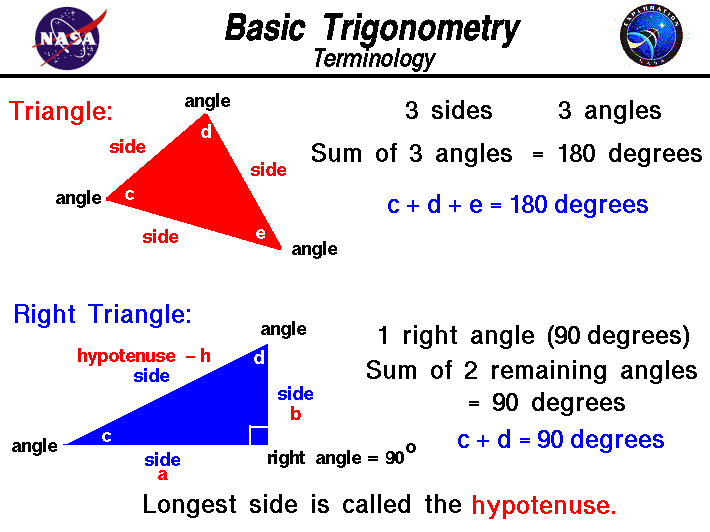+ Text Only Site
+ Non-Flash Version
+ Contact GlennTo better understand certain problems involving rockets and propulsion it is necessary to use some mathematical ideas from trigonometry, the study of triangles. Most people are introduced to trigonometry in high school. There are many complex parts to trigonometry, but on this page we are concerned chiefly with definitions and terminology. We start with a general triangle. A triangle is a closed shape having three sides and three internal angles. The sum of the three angles of any triangle is 180 degrees. If we label the angles of a triangle c, d, and e, then: c + d + e = 180 degrees There are two ways to measure the angles inside a triangle. One way is to measure the angle in degrees, where 360 degrees equals a complete circle. The other way is measure the angle in radians, where 2 pi radians equals a complete circle. Therefore; 360 (degrees) = 2 * pi (radians) 1 degree = .01745 radians 1 radian = 57.2957 degrees A right triangle is a special case of the general triangle with one of its angles equal to 90 degrees. A 90 degree angle is called a right angle and that is where the right triangle gets its name. The right triangle has some special properties which are very useful for solving problems. The sum of the three angles of a right triangle is equal to 180 degrees and one of the angles equals 90 degrees. Then the sum of the other two angles is also 90 degrees. For a right triangle: c + d = 90 degrees = pi/2 radians The important factor here is that if we know (or measure) one angle of a right triangle,we automatically know the value of the other angle. If we know the value of d, then c = 90 - d To describe a triangle in general, we need to know the value of two angles; for a right triangle we only need to know (or measure) one angle. Another important piece of information relates the size of the sides of a right triangle. We call the side of the right triangle opposite from the right angle the hypotenuse. It is the longest side of the three sides of the right triangle. The word "hypotenuse" comes from two Greek words meaning "to stretch", since this is the longest side. We will label the hypotenuse with the symbol h and we will label the other two sides a and b. Regardless of the size of the hypotenuse, the ratio of the size of side a to the hypotenuse h depends only on the size of the angle between the side and the hypotenuse. The value of the ratio is a function of the angle and is given the name cosine of the angle. On the figure, cos(c) = a / h Because of the relations of the angles of a right triangle, we can define another function of the angle, called the sine of the angle, which relates side b and the hypotenuse: sin(c) = b / h The key point here is that if we measure one angle, we know the value of all three angles in a right triangle. And if we additionally measure one side, we can use these trigonometric functions to determine the length of all three sides. We can determine 5 pieces of information (2 angles and 3 sides) by making only two measurements. An additional relation exists between the sides of a right triangle. If we draw a square on the hypotenuse, and a square on each of the two sides, the area of the square on the hypotenuse is equal to the sum of the squares on the sides. This is called the Pythagorean Theorem and has been know since ancient times: h^2 = a^2 + b^2 The Pythagorean Theorem can be used with the trigonometric functions to determine the size of all the sides of a right triangle. Activities: Guided ToursTrigonometry:Flight Equations:Maximum Altitude:Related Sites: Rocket Index Rocket Home Beginner's Guide Home+ Inspector General Hotline + Equal Employment Opportunity Data Posted Pursuant to the No Fear Act + Budgets, Strategic Plans and Accountability Reports + Freedom of Information Act + The President's Management Agenda + NASA Privacy Statement, Disclaimer, and Accessibility CertificationEditor: Tom Benson NASA Official: Tom Benson Last Updated: Jun 12 2014 + Contact Glenn